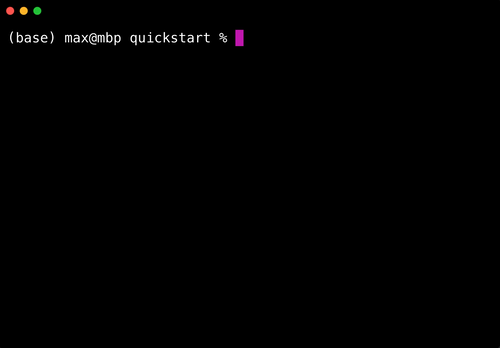# Home

###`mummify` is a version control tool for machine learning. It's simple, fast, and designed for model prototyping.

### Quickstart### Usage

Add `mummify.log(<string>)` to the bottom of a machine learning model:

``````from sklearn.datasets import load_wine
from sklearn.neighbors import KNeighborsClassifier

import mummify

X, y = data.data, data.target

model = KNeighborsClassifier(n_neighbors=4)
model.fit(X, y)
accuracy = round(model.score(X, y), 4)

mummify.log(f'Accuracy: {accuracy}')
``````

Run the model at the command line:

``````python model.py
``````

Edit the model to implement another algorithm:

``````...
model = LogisticRegression()
model.fit(X, y)
accuracy = round(model.score(X, y), 4)

mummify.log(f'Accuracy: {accuracy}')
``````

Inspect model history at the command line with:

``````mummify history
``````

And peek at the logged messages at the command line with:

``````cat mummify.log
``````

Switch to an earlier version of the model:

``````mummify switch <id>
``````

`mummify` will persist snapshots and the `mummify.log` file between switches.

### Installation

``````pip install mummify
``````

### Contribute

For feature requests or bug reports, please use Github Issues